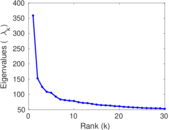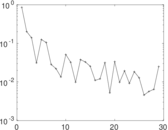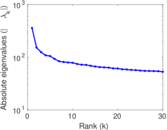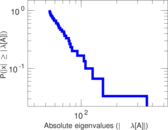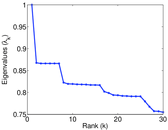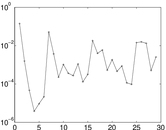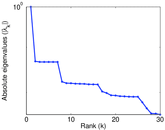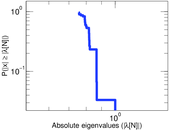This is the bipartite network of users commenting on movies, from the Swedish movie rating website Filmtipset.se. The nodes of the network are the users and the movie. Each link connects a user with a movie, and denote a single comment by that users on that movie. Multiple link between the same user–movie pair are allowed. Each link is annotated with the timestamp of the comment.

 Code `Fc` Internal name `filmtipset_comment` Name Filmtipset comments Data source http://www.dai-labor.de/camra2010/datasets/ Availability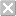Dataset is not available for download Consistency checkDataset passed all tests Category Interaction network Dataset timestamp 2010 Node meaning User, movie Edge meaning Comment Network formatBipartite, undirected Edge typeUnweighted, multiple edges Temporal dataEdges are annotated with timestamps

## Statistics

 Size n = 75,360 Left size n1 = 29,530 Right size n2 = 45,830 Volume m = 1,266,753 Unique edge count m̿ = 1,204,831 Wedge count s = 493,367,139 Claw count z = 204,725,098,142 Cross count x = 136,282,597,862,835 Square count q = 2,724,858,975 4-Tour count T4 = 23,774,988,546 Maximum degree dmax = 5,659 Maximum left degree d1max = 5,659 Maximum right degree d2max = 2,252 Average degree d = 33.618 7 Average left degree d1 = 42.897 2 Average right degree d2 = 27.640 3 Fill p = 0.000 890 252 Average edge multiplicity m̃ = 1.051 39 Size of LCC N = 75,194 Diameter δ = 9 50-Percentile effective diameter δ0.5 = 3.297 78 90-Percentile effective diameter δ0.9 = 4.305 01 Median distance δM = 4 Mean distance δm = 3.749 21 Gini coefficient G = 0.803 195 Balanced inequality ratio P = 0.171 261 Left balanced inequality ratio P1 = 0.169 335 Right balanced inequality ratio P2 = 0.144 105 Power law exponent γ = 1.578 01 Tail power law exponent γt = 1.581 00 Tail power law exponent with p γ3 = 1.581 00 p-value p = 0.000 00 Left tail power law exponent with p γ3,1 = 2.041 00 Left p-value p1 = 0.000 00 Right tail power law exponent with p γ3,2 = 1.631 00 Right p-value p2 = 0.000 00 Degree assortativity ρ = −0.186 575 Degree assortativity p-value pρ = 0.000 00 Algebraic connectivity a = 0.196 326 Controllability C = 40,256 Relative controllability Cr = 0.534 183

## Plots

### Fruchterman–Reingold graph drawing### Degree distribution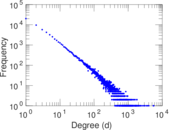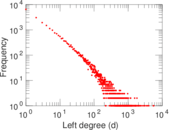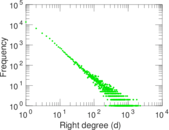### Cumulative degree distribution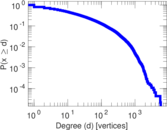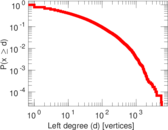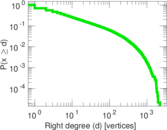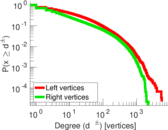### Lorenz curve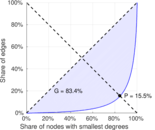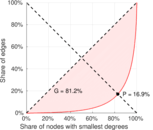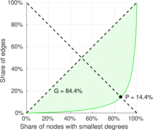### Spectral distribution of the adjacency matrix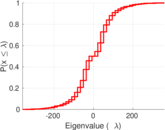### Spectral distribution of the normalized adjacency matrix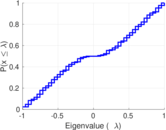### Spectral distribution of the Laplacian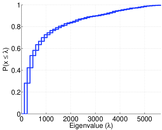### Spectral graph drawing based on the adjacency matrix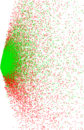### Spectral graph drawing based on the Laplacian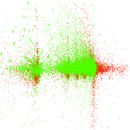### Spectral graph drawing based on the normalized adjacency matrix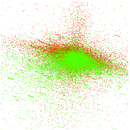### Degree assortativity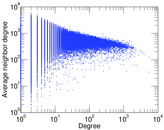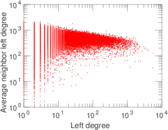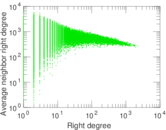### Zipf plot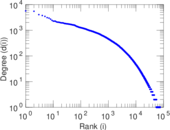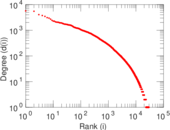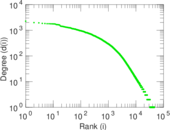### Hop distribution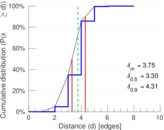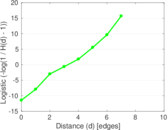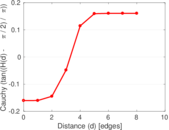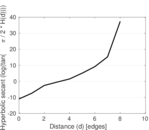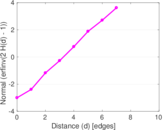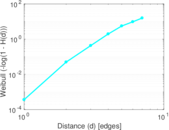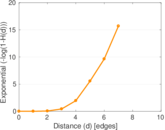### Delaunay graph drawing### Edge weight/multiplicity distribution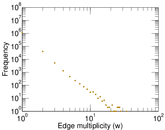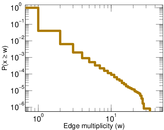### Temporal distribution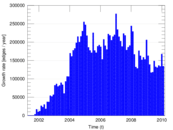### Temporal hop distribution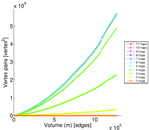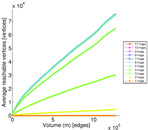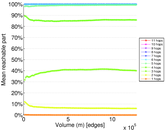### Diameter/density evolution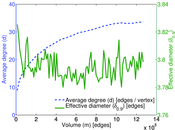### Inter-event distribution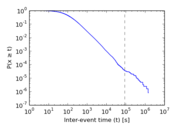### Node-level inter-event distribution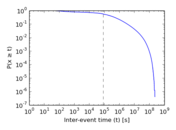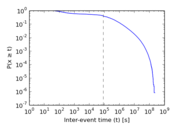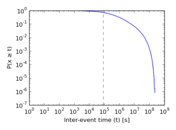### Matrix decompositions plots